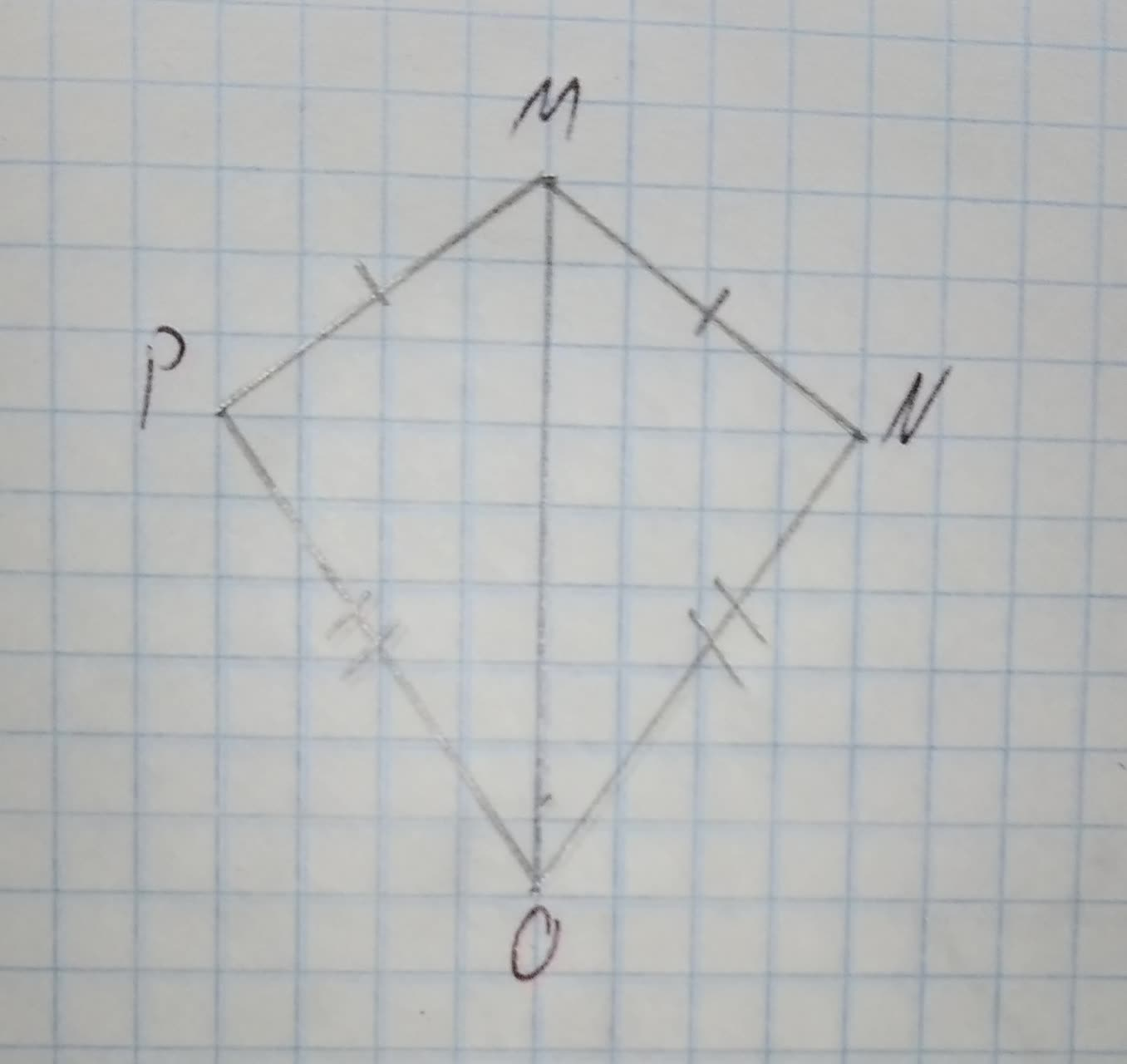# Write a two-column proof. Given: bar(MN) ~= bar(MP), bar(NO) ~= bar(PO) Prove: /_N ~= /_P 12210203572.jpgElleanor Mckenzie 2020-11-12 Answered
Write a two-column proof.
Given: $\stackrel{―}{MN}\stackrel{\sim }{=}\stackrel{―}{MP},\stackrel{―}{NO}\stackrel{\sim }{=}\stackrel{―}{PO}$
Prove: $\mathrm{\angle }N\stackrel{\sim }{=}\mathrm{\angle }P$You can still ask an expert for help

## Want to know more about Congruence?

• Questions are typically answered in as fast as 30 minutes

Solve your problem for the price of one coffee

• Math expert for every subject
• Pay only if we can solve itsaiyansruleA
Step 1
Given:
$\stackrel{―}{MN}\stackrel{\sim }{=}\stackrel{―}{MP},\stackrel{―}{NO}\stackrel{\sim }{=}\stackrel{―}{PO}$To prove:
$\mathrm{\angle }N\stackrel{\sim }{=}\mathrm{\angle }P$
Step 2
Thus, The reflexive property of congruence,
$\stackrel{―}{MO}\stackrel{\sim }{=}\stackrel{―}{MO}$
Now Triangles are congruent if all three sides in one triangle are congruent to the corresponding sides in the other.
By SSS congruence,
$\mathrm{△}MNO\stackrel{\sim }{=}\mathrm{△}MPO$
Corresponding parts of congruent triangle (CPCT).
Their corresponding sides are equal and angles are equal.
Therefore, $\mathrm{\angle }N\stackrel{\sim }{=}\mathrm{\angle }P$